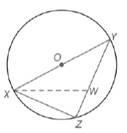Chapter 6.1, Problem 43E### Elementary Geometry for College St...

6th Edition
Daniel C. Alexander + 1 other
ISBN: 9781285195698

#### Solutions

Chapter
Section### Elementary Geometry for College St...

6th Edition
Daniel C. Alexander + 1 other
ISBN: 9781285195698
Textbook Problem
121 views

# In ⊙ O , O Y = 5 and X Z = 6. If X W ¯ ≅ W Y ¯ , find W Z .To determine

To calculate:

The WZ by using the provided figure.

Explanation

Given: In O, OY=5, and XZ=6 if XW¯WY¯

Pythagorean Theorem:

In a right triangle XYZ, (XY)2+(YZ)2=(XZ)2.

Calculation:

We need to find WZ.

Let WZ=y and x represent the measure of the congruent segments XW¯WY¯.

Since, OY=5 is a radius of circle O.

We know that, the diameter is double the radius of the circle.

Thus, XY=10.

Since, XYZ and XWZ are right triangles.

Thus, by using the Pythagorean theorem for XYZ triangle.

### Still sussing out bartleby?

Check out a sample textbook solution.

See a sample solution

#### The Solution to Your Study Problems

Bartleby provides explanations to thousands of textbook problems written by our experts, many with advanced degrees!

Get Started

#### Find more solutions based on key concepts